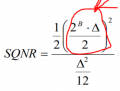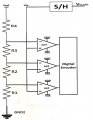# SQNR of IDIAL vs non idial FLASH ADC

#### yef smith

Joined Aug 2, 2020
74
Hello, I have a formula for SQNR when we tale Half of the full scale and we do it for sinus input for some reason
but in FLASH ADC shown bellow we dont divide by half the FULL SCALE for SNQR.
Full SCALE=V_max-Vmin of the values possible to be recieved by the ADC
So when we divide by 2 and when we dont?
Thanks.#### andrewmm

Joined Feb 25, 2011
638

#### yef smith

Joined Aug 2, 2020
74
I dont understand the definition of SQNR, We have two ranges ,1. is the range pk-pk of the voltages which ADC can handle and the other is the range of the input pk-pk.
Which one should i use for SQNR?

#### bogosort

Joined Sep 24, 2011
519
Hello, I have a formula for SQNR when we tale Half of the full scale and we do it for sinus input for some reason
but in FLASH ADC shown bellow we dont divide by half the FULL SCALE for SNQR.
Full SCALE=V_max-Vmin of the values possible to be recieved by the ADC
So when we divide by 2 and when we dont?
Thanks.
View attachment 218999
Two different ways of looking at the same thing. From the perspective of the ADC, the full-scale voltage range is Vmax - Vmin, regardless of what the input signal is.

Now, consider the voltage range for a sinusoid of amplitude A. Its peak voltage is A, but its full-scale (peak-to-peak) range is 2A. The SQNR of an ADC is specified in terms of rms voltages (or, equivalently, average power). So, to calculate the SQNR of an ADC with a sinusoidal input, the peak-to-peak range of the sinusoid must be converted to an rms value. This is done by dividing the Vpp value by $$2 \sqrt{2}$$, which is where the division by 2 comes in.

For example, suppose you have a single-ended, unipolar 8-bit ADC with a full-scale range of 0 to 10 V and you want to digitize a sine of 5 V amplitude (which is 10 V peak-to-peak). You could apply a 5 V dc offset to the sine, shifting the sine's "zero" value to 5 V. Then, when the sine is at its positive peak (+5 V) the ADC sees 10 V, and when the sine is at its negative peak (-5 V) the ADC sees 0 V.

In your equation, the full-scale voltage is given by the product of the full-scale codeword and the quantization step size: $V_{FS} = 2^B \Delta$ With respect to the SQNR equation, this needs to be an rms voltage (hence the division by square root of 2); with respect to the sine, it's a full-scale (peak-to-peak) voltage (hence the division by 2).

In other words, $V_{\text{rms}(\text{sine})} = \sqrt{\frac{1}{2} \left( \frac{2^B \Delta}{2} \right)^2} = \frac{V_{pp}}{2 \sqrt{2}}$
This rms value only applies to sinusoidal inputs.

#### bogosort

Joined Sep 24, 2011
519
I dont understand the definition of SQNR, We have two ranges ,1. is the range pk-pk of the voltages which ADC can handle and the other is the range of the input pk-pk.
Which one should i use for SQNR?
SQNR is the ratio between the signal's power (as seen by the ADC) and the ADC's quantization noise power, which depends on the signal, so you always use the input signal's peak-to-peak value. Remember, even with the same ADC, different signals can have different SQNRs.